# Scheme (mathematics)

Schemes, and functions between them, are the principal objects of study in modern algebraic geometry. Algebraic geometry began as the study of varieties, geometric figures described by polynomial equations with coefficents in a field. The geometric properties of an affine variety are reflected in algebraic properties in its ring of functions, which is the quotient of a polynomial ring. These algebraic properties can be defined in the context of arbitrary commutative rings, and affine schemes are the corresponding geometric objects. A general scheme is a geometric object which looks like an affine scheme in a neighborhood of every point.

Schemes have superseded varieties as the main objects of interest in algebraic geometry for several reasons: they give a uniform way to treat all previous disparate definitions of varieties, including affine, projective, quasi-projective, and abstract varieties, and there is a huge diversity of schemes that are not classical varieties. Also, the theory of varieties is most successful when the points on the varieties have values in an algebraically closed field. By contrast, important problems in arithmetic geometry involve studying arithmetic properties of points on varieties, which cannot be done by working over an algebraically closed field. Schemes have proven to be effective at overcoming this difficulty.

The theory of schemes was pioneered by Alexander Grothendieck. The foundations of scheme theory were initially organized in Grothendieck's multi-volume work Éléments de Géométrie Algébrique with the assistance of Jean Dieudonné.

A number of technical definitions and procedures are outlined in the glossary of scheme theory.

## The Category of Schemes

A scheme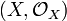$(X,\mathcal{O}_X)$ consists of a topological space X together with a sheaf$\mathcal{O}_X$ of rings (called the structural sheaf on X) such that every point of X has an open neighborhood U such that the locally ringed space$(U,\mathcal{O}_X\vert_U)$ is isomorphic to an affine scheme.

Projective schemes constitute an important class of schemes, especially for the study of curves.

The category of schemes is defined by taking morphisms of schemes to be morphisms of locally ringed spaces. Many kinds of morphisms of schemes are characterized affine-locally, in the sense that

## Gluing Properties

The notion of "gluing" is one of the central ideas in the theory of schemes. Let S be a scheme, and$(X_i)_{i\in I}$ a family of S-schemes. If we're given families$(X_{ij})_{j\in I}$ and S-isomorphisms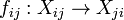$f_{ij}:X_{ij}\to X_{ji}$ such that: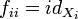$f_{ii}=id_{X_i}$,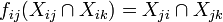$f_{ij}(X_{ij}\cap X_{ik})=X_{ji}\cap X_{jk}$, and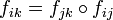$f_{ik}=f_{jk}\circ f_{ij}$ on$X_{ij}\cap X_{ik}$ for all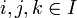$i,j,k\in I$, then there is an S-scheme X together with S-immersions$g_i:X_i\to X$ such that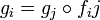$g_i=g_j\circ f_ij$ on Xij and so that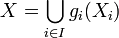$X=\bigcup_{i\in I} g_i(X_i)$. This scheme X is called the gluing over S of the Xi along the Xij.

The S-scheme X is universal for the property above: i.e., for any S-scheme Z and family of morphisms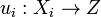$u_i:X_i\to Z$ such that$u_i=u_j\circ f_{ij}$ on Xij, there is a unique morphism$u:X\to Z$ such that$u_i=u\circ g_i$. Moreover, if Z is a scheme, then giving a morphism$u:Z\to X$ is equivalent to giving an open covering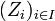$(Z_i)_{i\in I}$ of Z and morphisms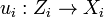$u_i:Z_i\to X_i$ such that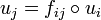$u_j=f_{ij}\circ u_i$ on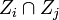$Z_i\cap Z_j$.

## Morphisms of SchemesSome content on this page may previously have appeared on Citizendium.